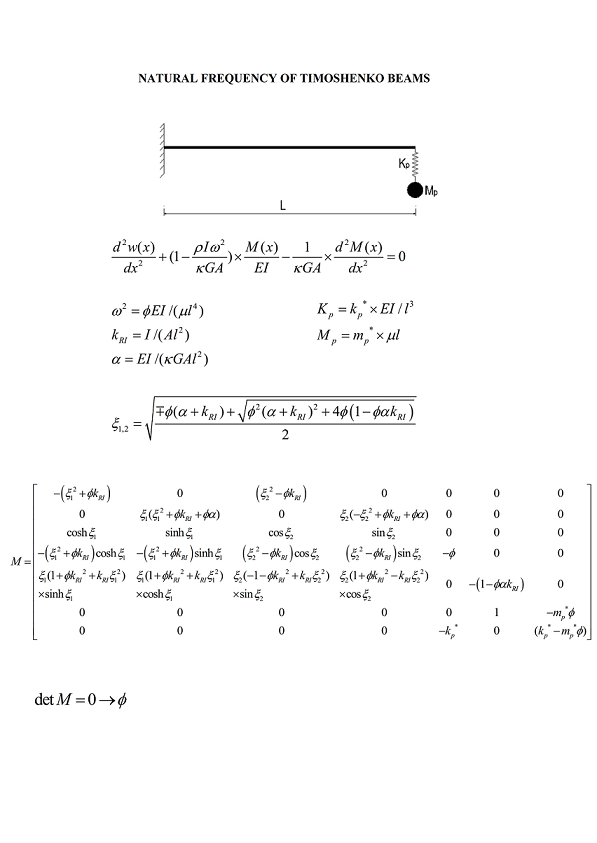Preprint Article Version 1 Preserved in Portico This version is not peer-reviewed

# An Exact Solution to the Free Vibration Analysis of a Uniform Timoshenko Beam Using an Analytical Approach

Version 1 : Received: 22 January 2021 / Approved: 25 January 2021 / Online: 25 January 2021 (14:09:44 CET)

How to cite: Fogang, V. An Exact Solution to the Free Vibration Analysis of a Uniform Timoshenko Beam Using an Analytical Approach . Preprints 2021, 2021010501 (doi: 10.20944/preprints202101.0501.v1). Fogang, V. An Exact Solution to the Free Vibration Analysis of a Uniform Timoshenko Beam Using an Analytical Approach . Preprints 2021, 2021010501 (doi: 10.20944/preprints202101.0501.v1).

## Abstract

This study presents an analytical solution to the free vibration analysis of a uniform Timoshenko beam. The Timoshenko beam theory covers cases associated with small deflections based on shear deformation and rotary inertia considerations. A material law combining bending, shear, curvature, and natural frequency is presented. This complete study is based on this material law and closed-form solutions are found. The free vibration response of single-span systems, as well as that of spring-mass systems, is analyzed. Closed-form formulations of matrices expressing the boundary conditions are presented; the natural frequencies are determined by solving the eigenvalue problem. First-order dynamic stiffness matrices in local coordinates are determined. Finally, second-order analysis of beams resting on an elastic Winkler foundation is conducted.## Subject Areas

Timoshenko beam; rotary inertia; bending shear curvature natural frequency relationship; spring mass system vibration; closed-form solutions; first-order dynamic stiffness matrix; second-order vibration analysis

Views 0